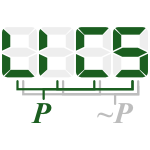`Used together with:`
linear (10)
equat (9)
system (6)
solv (5)
solut (4)

## Stem diophantin\$ (all stems)

### 13 papers:CAV-2008-JainCG #composition #equation #linear #performance
Efficient Craig Interpolation for Linear Diophantine (Dis)Equations and Linear Modular Equations (HJ, EMC, OG), pp. 254–267.CIAA-2003-XieLD #complexity #equation #linear #problem #using
New Complexity Results for Some Linear Counting Problems Using Minimal Solutions to Linear Diophantine Equations (GX, CL, ZD), pp. 163–175.ICALP-2003-XieDI #equation #infinity #polynomial #verification
A Solvable Class of Quadratic Diophantine Equations with Applications to Verification of Infinite-State Systems (GX, ZD, OHI), pp. 668–680.STOC-1999-Rojas #complexity #geometry #on the
On the Complexity of Diophantine Geometry in Low Dimensions (Extended Abstract) (JMR), pp. 527–536.ALP-PLILP-1998-AjiliL #constraints #linear
Integrating Constraint Propagation in Complete Solving of Linear Diophantine Systems (FA, HCRL), pp. 463–480.RTA-1997-TomasF #equation #geometry #linear #using
Solving Linear Diophantine Equations Using the Geometric Structure of the Solution Space (APT, MF), pp. 269–283.RTA-1995-AbdulrabM #equation #linear
General Solution of Systems of Linear Diophantine Equations and Inequations (HA, MM), pp. 339–351.ICLP-1993-Contejean #constraints #incremental #linear
Solving Linear Diophantine Constraints Incrementally (EC), pp. 532–549.STOC-1992-VenkatesanR #matrix #problem
Average Case Intractability of Matrix and Diophantine Problems (Extended Abstract) (RV, SR), pp. 632–642.RTA-1991-Pottier #algorithm #bound #linear
Minimal Solutions of Linear Diophantine Systems: Bounds and Algorithms (LP), pp. 162–173.LICS-1990-BoudetCD #algorithm #equation #unification
A New AC Unification Algorithm with an Algorithm for Solving Systems of Diophantine Equations (AB, EC, HD), pp. 289–299.RTA-1989-AbdulrabP #equation #linear #word
Solving Systems of Linear Diophantine Equations and Word Equations (HA, JPP), pp. 530–532.ICALP-1984-Schonhage #algorithm #integer #reduction
Factorization of Univariate Integer Polynomials by Diophantine Aproximation and an Improved Basis Reduction Algorithm (AS), pp. 436–447.

Bibliography of Software Language Engineering in Generated Hypertext (BibSLEIGH) is created and maintained by Dr. Vadim Zaytsev.
Hosted as a part of SLEBOK on GitHub.